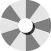### My Cart

Close

F r e e   S h i p p i n g   O n   A l l   O r d e r s   O v e r      \$ 1 2 0                F o r   A   L i m i t e d   T i m e   O n l y                    U p   T o   50%   O f f  S t o r e w i d e

F r e e   S h i p p i n g   O n   A l l   O r d e r s   O v e r      \$ 1 2 0                F o r   A   L i m i t e d   T i m e   O n l y                    U p   T o   50%   O f f  S t o r e w i d e

F r e e   S h i p p i n g   O n   A l l   O r d e r s   O v e r      \$ 1 2 0                F o r   A   L i m i t e d   T i m e   O n l y                    U p   T o   50%   O f f  S t o r e w i d e

# Exchange Policy

#### Explore

At We Shop Fashion, we offer Exchanges up to 30 days.

The product must be in the exact condition you bought it in, meaning unworn with the tags.

Ship it back to us and send an email to reg.liyanage@yortongroup.com

After we receive the item and examine its condition, we will let you know and send you a Gift Card via email. (check your junk folder)

Spin to win